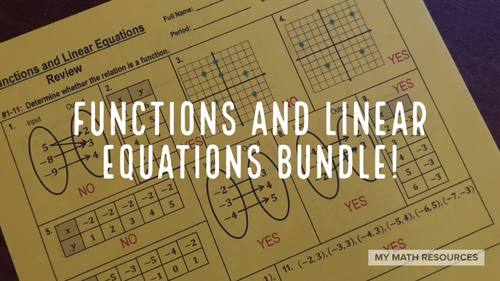# Functions and Linear Equations Quiz & Worksheet

Rated 4.85 out of 5, based on 26 reviews
26 Ratings;
6th - 9th
Subjects
Resource Type
Formats Included
• PDF
Pages
22 pages
Report this resource to TPT

### Description

Are you covering Functions and Linear Equations and need a little break?

This product contains a worksheet and an assessment.

Don't spend your valuable time making your own test or assessment...we took care of it for you!

Two Items Included. Want to buy them separately?

TOPICS BY QUESTION NUMBER:

• Questions 1-11: Tell whether the relation is a function from a table, graph, or input output line diagram.
• Questions 12-13: Identify the domain and range of the function.
• Questions 14-16: Determine whether the ordered pair is a solution to the equation.
• Questions 17-22: Write the equation in function form.
• Questions 23-24: Create a table of values. Then, graph the points on the coordinate plane.
• Questions 25-26: Graph the equation. Then, determine whether the equation is a function.

2 page Assessment:

TOPICS BY QUESTION NUMBER:

• Questions 1-5: Tell whether the relation is a function from a table, graph, or input output line diagram.
• Questions 6-7: Identify the domain and range of the function.
• Questions 8-9: Tell whether the ordered pair is a solution to the equation.
• Questions 10-11: Determine whether the ordered pair is a solution to the equation.
• Questions 12-13: Create a table of values. Then, graph the points on the coordinate plane.
• Questions 14-15: Graph the equation. Then, determine whether the equation is a function.
Total Pages
22 pages